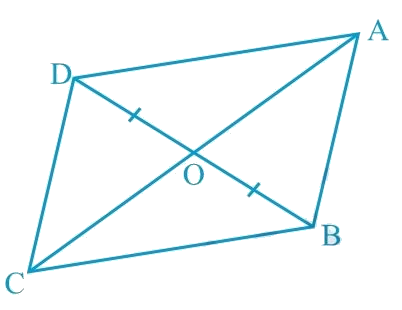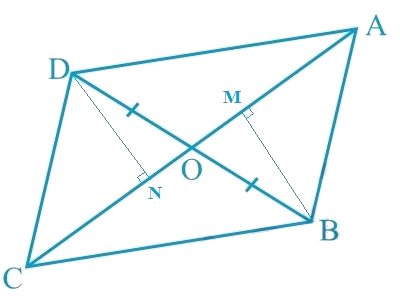In the verge of coronavirus pandemic, we are providing FREE access to our entire Online Curriculum to ensure Learning Doesn't STOP!

# Ex.9.3 Q6 Areas of Parallelograms and Triangles Solution - NCERT Maths Class 9

Go back to  'Ex.9.3'

## Question

In the given figure, diagonals $$AC$$ and $$BD$$ of quadrilateral $$ABCD$$ intersect at $$O$$ such that $$OB =OD$$. If $$AB = CD$$, then show that:

(i) $$\text { ar } (DOC) = \text {ar } (AOB)$$

(ii) $$\text { ar } (DCB) = \text {ar } (ACB)$$

(iii) $$DA \| CB \text { or } ABCD$$  is a parallelogram.

[Hint: From $$D$$ and $$B$$, draw perpendiculars to $$AC$$.]Video Solution
Areas Of Parallelograms And Triangles
Ex 9.3 | Question 6

## Text Solution

What is known?

Diagonals $$AC$$ and $$BD$$ of quadrilateral $$ABCD$$ intersect at $$O$$ such that  $$OB =OD.$$Also, $$AB = CD.$$

What is unknown?

How we can show that

(i) $$ar (DOC) = ar (AOB)$$

(ii) $$ar (DCB) = ar (ACB)$$

(iii) $$DA || CB$$ or $$ABCD$$ is a parallelogram.

Reasoning:

We can draw perpendicular from vertices $$B$$ and $$D$$ on diagonal $$AC$$ which will help us to make triangles congruent and we know that congruent triangles are always equal in areas. If two triangles have the same base and equal areas, then these will lie between the same parallels. If in a quadrilateral one pair of opposite sides is equal and the other pair of opposite sides is parallel then it will be a parallelogram.

Steps:Let us draw $$DN \perp AC$$ and $$BM \perp AC$$.

(i) In $$\Delta DON$$ and $$\Delta BOM$$,

\begin{align} & \angle DNO = \angle BMO = 90^{\circ}\end{align} (By construction)

\begin{align} & \angle DON = \angle BOM\; \end{align} (Vertically opposite angles are equal)

\begin{align} & OD = OB\;\end{align} (Given)

By AAS congruence rule,

\begin{align} \Delta DON & \cong \Delta BOM \\ DN &= BM \; \ldots (1)\end{align}

We know that congruent triangles have equal areas.

$\text{ar} (\Delta DON)\!=\!\text{ar} (\Delta BOM) \ldots (2)$

In $$\Delta DNC$$ and $$\Delta BMA$$,

\begin{align} & \angle DNC = \angle BMA = 90^{\circ} \;\end{align} (By construction of perpendicular bisectors)

\begin{align} & CD = AB \;\end{align} (Given)

$$DN = BM$$ [Using Equation (1)]

NOTE: RHS Congruence rule illustrates that, if the hypotenuse and one side of right angled triangle are equal to the corresponding hypotenuse and one side of another right angled triangle; then both the right angled triangle are said to be congruent.

\begin{align} \Delta DNC &\cong \Delta BMA\\text {RHS congru}&\text{ence rule}) \\ \text {ar} (\Delta DNC) &= \text {ar} (\Delta BMA) \ldots(3) \end{align} On adding Equations (\(2) and ($$3$$), we obtain

$\left[ \begin{array}{l}{\rm{ar}}(\Delta DON) + \\{\rm{ar}}(\Delta DNC)\end{array} \right]\!\!=\!\!\left[ \begin{array}{l}{\rm{ar}}(\Delta BOM) + \\{\rm{ar}}(\Delta BMA)\end{array} \right]$

Therefore, $$\text{Area }(\Delta DOC) = \text{Area }(\Delta AOB)$$

(ii) We obtained,

\begin{align}& \text{Area }(\Delta DOC) = \text{Area }(\Delta AOB) \end{align}

$\therefore\left[ \begin{array}{l}{\rm{ar}}(\Delta DOC) + \\{\rm{ar}}(\Delta OCB)\end{array} \right]\!\!=\!\!\left[ \begin{array}{l}{\rm{ar}}(\Delta AOB) + \\{\rm{ar}}(\Delta OCB)\end{array} \right]$

Adding Area $$(\Delta OCB)$$ to both sides

\begin{align} & \therefore \text {ar} (\Delta DCB) = \text {ar} (\Delta ACB) \end{align}

(iii) We obtained,

$\text{ar}(\Delta DCB)\!\!=\!\!\text{ar}(\Delta ACB)$

If Two triangles having the same base (or equal bases) and equal areas lie between the same parallels.

$\therefore DA \|CB \; \ldots (4)$

In quadrilateral $$ABCD$$, one pair of opposite sides is equal $$(AB = CD)$$ and the other pair of opposite sides is parallel $$(DA\|CB)$$.

Therefore, $$ABCD$$ is a parallelogram.

Video Solution
Areas Of Parallelograms And Triangles
Ex 9.3 | Question 6